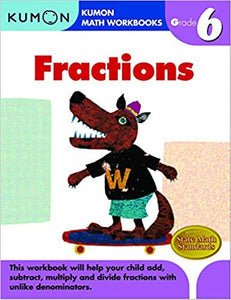• \$5.96
Unit price per
Shipping calculated at checkout.

Most grade-school workbooks try to cover the full range of topics in one workbook, and as a result do not dedicate enough pages to important mathematical concepts. Kumon's research has found, however, that children find learning easier when they tackle one skill set at a time. With these findings in mind, we designed our newest line of workbooks to help children with one particular mathematical skill set per book.

In this workbook, children gain foundational skills for calculating fractions. Sections on greatest common factor and lowest common multiple prepare children for adding and subtracting fractions with unlike denominators, as well as multiplying and dividing fractions and mixed numbers.

Topics Covered in this Book:

• Reducing fractions to lowest possible denominator
• Factors and multiples
• Greatest common factor (GCF)
• Lowest common multiple (LCM)
• Addition & subtraction of fractions with unlike denominators
• Multiplication & division of fractions and mixed numbers
• Changing decimals into fractions
• Calculations with three fractions

8½ x 11 inches. paperback. 96 pages. full color.

* Due to pandemic delays, please allow 2 weeks for items to ship